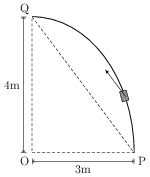# Kinetic and Potential Energy

## Problems from IIT JEE

Problem (IIT JEE 2014):Consider an elliptically shaped rail PQ in the vertical plane with $\mathrm{OP}={3}\;\mathrm{m}$ and $\mathrm{OQ}={4}\;\mathrm{m}$. A block of mass 1 kg is pulled along the rail from P to Q with a force of 18 N, which is always parallel to line PQ (see figure). Assuming no frictional losses, the kinetic energy of the block when it reaches $Q$ is $(n\times10)$ Joules. The value of $n$ is (Given $g={10}\;\mathrm{m/s^2}$.),

Solution: Consider a small displacement $\mathrm{d}\vec{s}$ along the elliptical path. Resolve $\mathrm{d}\vec{s}$ in directions parallel and perpendicular to $\vec{F}$, say $\mathrm{d}\vec{s}_{\parallel }$ and $\mathrm{d}\vec{s}_{\perp}$. The work done by $\vec{F}$ for the path from P to Q is, \begin{align} \label{bxb:eqn:1} W=\int\vec{F}\cdot\mathrm{d}\vec{s}=\int\vec{F}\cdot\mathrm{d}\vec{s}_{\parallel} = F\int\mathrm{d}\vec{s}_{\parallel}=18(5)={90}\;\mathrm{J}. \end{align} By work-energy theorem, \begin{align} \label{bxb:eqn:2} W=\Delta K+\Delta U, \end{align} where $\Delta U=U_f-U_i$ is the change in the potential energy and $\Delta K$ is the change in the kinetic energy. From above equations, \begin{align} \Delta K=W-mgh=90-1(10)4={50}\;\mathrm{J}.\nonumber \end{align}